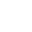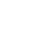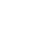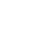Home » Teaching Place Value? How NOT to do it the Wrong Way!

# Teaching Place Value? How NOT to do it the Wrong Way!

Find out how to teach place value developmentally and appropriate to the grade with lesson ideas and a FREE Place Value Game!

I can still see Mrs. Pink my high school math teacher (I was her T.A.) explaining to her 1st-period class why they might want to learn how to write a check correctly (this was back in the 70s, so everyone had to learn how to write a check!). Do you really want to put that decimal point just anywhere? Not understanding place value can have serious financial consequences!

But if understanding place value means correctly interpreting the value of a digit based on its place in a number, then just having students use a place value chart is probably not enough!

Teaching place value involves a lot of moving parts.  Students need to have certain other concepts in place before they can genuinely internalize the value of digits.

Teaching place value is essential because it is the last brick in building number sense in elementary students.  In this post, I will explain how place value develops over the grades. I will also show why teaching place value must be learned concretely and not abstractly so that your students develop a solid conceptual understanding and not a superficial knowledge of place value.

Are you wondering how to teach place value in a distance learning model? Read more about how to teach place value virtually online.

READ TO THE END TO FIND OUT HOW TO RECEIVE A FREE PLACE VALUE GAME!

## The Big Picture Progression for Teaching Place Value

If you are teaching using the Common Core State Standards for Mathematics, you know that the standards are coherent.  One standard is developed in-depth across many grade levels. Place value is one of those standards.

Beginning in Kindergarten, students learn that the teen numbers (11 – 29) are made up of a 10 + x.  So 18 can be seen as  10 and 8 (that’s why it’s essential to know how to make 10!)  Students in first grade continue with this as they learn numbers up to 120.  They learn that tens and ones make up two-digit numbers.  They learn to bundle 10 ones as a ten.  These standards all lay the essential foundations of place value:  the digit to the left is 10 times more than the digit to the right or conversely, the numbers to the right are one-tenth as much as the numbers to the left.

## Place Value Gets Real!

In second grade, teaching place value for numbers up to 1,000 is now paramount.  Students must not leave second grade without an understanding of the value of digits as units, tens, and hundreds.  Not only will they use place value to identify multidigit numbers, but also to add and subtract and eventually multiply and divide.

By third grade, students may need a review of place value but should have a solid understanding of place value up to 1,000.  Third grade is when fractions are introduced as numbers in a standard form.  Knowing whole numbers and values is necessary for understanding the fractions that fall in between whole numbers.

In fourth and fifth grades, place value goes in another direction:  decimals.  Yes, the place value of whole numbers is expanded into the hundred thousand but also into the thousandths for decimals. By the end of fifth grade, most students should have a decent if not a solid conceptual understanding of place value for whole numbers, fractions, and decimals.

READ TO THE END TO FIND OUT HOW TO RECEIVE A FREE PLACE VALUE GAME!

## Manipulatives for Teaching Place Value

Students need to see place value concretely, not abstractly with digits on a place value chart.  So it matters which manipulatives you use.  When teaching place value, you can categorize manipulatives as groupable or pre-grouped.

## Place Value Manipulatives

Groupable ManipulativesPre-Grouped Manipulatives
unifix or linker cubesbeans glued to a stick
paperclipsplace value disks or chips
strawsbase 10 blocks
countersLegos®

It’s critical to start with the groupable manipulatives first. Let students go through the motion of bundling and unbundling groups of ten or groups of one hundred.  Most teachers in Kindergarten and first grade will use base 10 blocks to teach place value. But these are not appropriate at these grades because the base 10 blocks can not be ungrouped. Yes, they can be traded, but that is not the same.

## Bundling and Trading in Place Value

In Kindergarten and first grade, it is critical that students bundle.  Whether they bundle by putting a rubber band around straws, connecting Unifix cubes or grouping objects into a cup, the act of bundling builds that conceptual understanding of 10 times more.  It also teaches students to unitize.  When they count the group, it’s counted by 10.  So it’s essential to use groupable manipulatives at these grade levels. Once students understand bundling, then begin the switch to base 10 blocks.

In second grade and up, base 10 blocks are more appropriate because students have built up that conceptual understanding of place value as 10 times more, and that one bundle of ten is ONE TEN.  Second graders begin bundling groups of tens to make 100. Second graders need a lot of practice for bundling those tens to make hundreds.  Base 10 blocks are appropriate to do this.

By third grade and up, students have internalized the idea of a group for 10 and 100.  They will use these to build numbers and place value to 1,000. More importantly, they will use them to add and subtract with regrouping.

FREE PLACE VALUE GAME!

Students in grades K – 3 should have many experiences to express numbers in many ways.  Students should practice representing the same number in various configurations.   See the example below.

By fourth and fifth grade, the base 10 blocks become decimals.  The thousand cube becomes tens, and the flat becomes one, while the rod becomes the tenths, and the unit cube becomes the hundredths.  That can be confusing! Here’s a video to explain it.

## Where does Teaching Place Value Begin?

It begins with counting. In Pre-K and Kindergarten, students are being taught to count.  Counting involves a lot of subskills and understandings: subitizing, tagging, one-to-one correspondence, hierarchical inclusion, verbal counting, skip counting, comparing numbers, and matching quantities to the standard form of the number.

From there, teaching place value (though it may not seem like it) is teaching Kinder and first graders the idea of composing and decomposing numbers and making a ten.  Making a ten is the first exposure to teaching place value formally.

## Bundling and Unbundling is Part of Teaching Place Value

Once students know how to make a ten, the next step is to teach students to count that ten as one unit – one ten!  When we do this, it is unitizing. I’ve written before about how unitizing is a big math idea.  The idea that you can bundle 10 individual units as one is a big math idea for little ones to wrap their heads around, especially after we’ve stressed the importance of one-to-one correspondence.  Now 10 becomes 1!

One very effective way to teach unitizing is through counting collections. Just have them count! They can count anything. The purpose here is for students to develop efficient systems of counting. They’ll realize that grouping by tens, and eventually, hundreds will help keep track of counting.  They’ll see that counting one object by hundreds or tens is a very efficient way to count large quantities.

## Counting Collections for Practicing Place Value

For long term gain, you want students counting! If you are not familiar with counting collections, check out this website.  Counting collections are a great way to get students to count in a meaningful and fun way.  Students can count anything!  Counting collections are great for math centers or small group work.  Students can record their collections in ten frames or by drawings or by writing the number.

What are objects you can count?

• buttons
• paper clips
• pencils
• beans
• seeds
• counters
• cubes
• tiles

My suggestion is to send a letter home to parents asking for donations of buttons, a bag of beans, nuts, and washers, a bag of macaroni, etc.  Students can count anything.  Put these materials into bags with a range of quantities depending on the needs of your students.  Let them record their findings onto a sheet of blank ten frames, hundred frames or some other recording sheet.

By the way, even upper elementary would benefit from counting.  Check out this article from the National Council of Teachers of Mathematics (NCTM) on counting collections for the upper grades, including choral counting.

## Some Ways for Teaching Place Value

When you’re teaching place value to students, please consider the following:

• student grade level and the specifics of the standard
• use of appropriate manipulatives to make the experience visual and concrete
• give the students time!

It takes time for students in K – 2 to internalize digit values because numbers by their nature are just symbolic representations of a quantity.  But when we start with the concrete so students can see and touch math, we can solidify their understanding.

Don’t also forget that when students are practicing with math tools such as base 10 blocks or other counting materials, they are using the math tools (Standard for Mathematical Practice 5) to look for and make use of place value structure (Standard for Mathematical Practice 7).  When students take a number represented in one form such as word form and try to reason abstractly and quantitatively (Standard for Mathematical Practice 2) to represent that number in other forms they are modeling mathematics (Standard for Mathematical Practice 4) because they are using math symbols (numbers, expanded form, base 10 units) to represent a quantity. As you can see, place value is a great way to have students think mathematically.

FREE PLACE VALUE GAME!

Check out this video about the Common Core State Standards for Place Value (with one of the authors of the Common Core!)

What follows is a Kindergarten, first and second grade-specific activity you can use to develop place value.

## Place Value in Kindergarten

In Kindergarten, the Common Core State Standard for Mathematics that relates to place value states: compose and decompose numbers from 11 – 19 into tens and ones by using objects or drawings and record as a drawing or equation.

With Unifix or linker cubes, students can build towers to get to 10.  Here’s a fun activity that will help with learning the teen numbers.  This activity is for three students playing together.  For this activity, you will need at least 20 – 30 Unifix cubes for each group of 3.

### Unifix or Linker Cube Activity

1. Students sit in a circle around a pile of Unifix cubes.
2. Taking turns, each grabs a handful with ONE hand.
3. Then each student counts how many cubes each one has.
4. Together the students decide which of the three students can make a ten by combining two of the handfuls. For example, if one of the students had 6 cubes and the other 4, that would make ten.  If a combination can’t be made, then everyone puts the cubes back and tries again with another handful.
5. Combine the handfuls that make a ten into a train or tower, then count the remaining hand amount.
6. Together the students count the total by saying “10 and ___ make ____.”  For example, after making the ten, there were 6 leftover Unifix cubes, they would say, “10 and 6 make 16.”
7. Then the students return all the cubes to the pile unlinked to play again.
8. Play repeats.

A variation of this is for students to work independently by grabbing a handful with both hands.  Then the student makes a ten train with the cubes.  Together with the remaining cubes, the student says 10 and ___ make ____.”  You can also have the students record their number on a recording sheet.

FREE PLACE VALUE GAME!

## Place Value in First Grade

In first grade, the Common Core State Standard for Mathematics that relates to place value states:  Understand that the two digits of a two-digit number represent amounts of tens and ones.

Bundling 10 units into one ten is part of this standard. Bundling is unitizing.  Here’s an activity to build bundling and understanding tens and ones.  For this activity, you will need one set of cards with numbers 11 – 99, bundled tens and cubes or counters or anything that can be counted as units.  You will also need to have a duplicate set of the number cards.

### Bundling Activity

1. Divide the class in half.  One half comes up to the front; the other half remains at their seats.
2. Pass out one number card to each child that is seated. For every card that you pass out, have the duplicate card set aside for the standing students.
3. Seated children use the bundles of ten and units to build the number on the card.  Then turn their card over face down.
4. Once all the seated students have built their numbers, give the standing students one of the duplicate cards.
5. The standing students now find their number that one of the students has built.  When they find their number, they stand behind the other student.
6. Once all students have found their number, the sitting students turn over their cards to see if they match the standing student’s card.
7. Before repeating for another turn, ask the sitting students how they knew how many tens and ones to use to build the number. Ask the standing students how they knew they found the matching number.
8. Standing students now switch with the sitting students and repeat the process.

Use counting collections to practice unitizing and build place value knowledge.  Refer above to the Place Value in Kindergarten for how to use counting collections.

Another factor to consider when teaching place value is to teach students to draw the bundles or base 10 blocks. For example, the rod representing ten is just a line while the cubes representing units or ones are just squares.  Drawing the base 10 blocks follows the CRA model of concrete-representational-abstract.

## Place Value in Second Grade

In second grade, the Common Core State Standard for Mathematics that relates to place value states: Understand that the three digits of a three-digit number represent amounts of hundreds, tens and ones.

Essentially, the second-grade standard is expanded to now include hundreds.  But to get to the hundreds, the students need to have a solid foundation with tens and ones.  This foundation should consist of the notion of bundling and unbundling a group of ten as well as, representing any number in many combinations:  43 can be 4 tens and 3 ones, but also 3 tens and 13 ones, or 2 tens and 23 ones or 1 tens and 33 ones or even 43 ones.

For second graders, we want them to bundle 10 groups of 10 to make one hundred.  Here’s an activity to practice bundling of tens to make hundreds. For this activity, you will need a set of cards 0 – 9 (2 of each for a total of 20 cards), base 10 blocks (units, rods, and flats) and a place value mat.  Students can play individually, in pairs, or a group.  The goal is to get to a certain number.  You can decide the number based on time allotted to play(play to 300, 400, 500, or more).

### Bundling Groups of Ten Activity

1. The student picks two number cards. The student decides what number to call.  For example, if I choose 5 and 2, it could be 52 or 25.  If my goal is to get to a certain, I will pick the greater number.
2. The student then builds the number using base 10 blocks on the place value mat.
3. If playing with other students, the others take their turns and do the same.
4. When a student has 10 units, the student must bundle to make a ten rod.  When the student has 10 rods, the student must bundle them for a flat or 100.
5. The first student to get to the designated number wins. Then the others are ranked based to see who has the next highest total, etc.

FREE PLACE VALUE GAME!

## Place Value Now Takes on New Forms

Second graders also represent numbers in other ways than just base 10 blocks.  They learn to express 3 digit numbers in standard form, expanded form, word form, and base 10 units (i. e., 236 has 2 hundreds, 3 tens and 6 ones).  A number can go from concrete to abstract depending on how it is represented, as shown in the chart below.

When students are learning digit values, we want them to transition from the concrete to the abstract slowly.  We don’t want to plug in digits into a place value chart and ask how many hundreds, tens and ones.  Instead, we want to work our way up to the abstract. When students represent numbers in the expanded form, they can see the digit values.  For example, 394 is the same as 300 + 90 + 4.  Each digit in that number is written as a value, so it is clear that the 3 is not just 3 but 300 and so forth.

### Ground the Place Value in the Concrete

Working in base 10 units, students can take that same number and say that the 3 is in the hundreds place, so it is 3 hundreds, 9 tens and 4 ones.  This is more abstract in representing the number because, in their mind, students have to visualize hundreds represented with 2 zeroes, tens with one zero and the ones as single digits.  One way to strengthen this visualization is to connect the expanded and standard forms with the base 10 units.

Going even to a more abstract representation is word form.  Word form can be problematic in that if a child is unable to read the words, then the point becomes moot.  But if the child can read the words, writing 394 in word form (three hundred ninety-four) can be challenging if it is misinterpreted.  For example, saying the word form for 394 might be interpreted as 30094 (three hundred then 94).

To help students learn to identify numbers and their values in word form, connect it to something less abstract such as the base 10 blocks or expanded form.

Finally, once students have a good understanding of digit values, you can transition to a place value chart.  By then, they should have internalized or be able to visualize the value of the digits by just looking at their place in the number.  So, if you start with a place value chart when teaching place value, you have started with the most abstract representation of place value.  That is why I would consider that to the wrong way to start.

## Resources for Teaching Place Value

Having taught second grade just recently, and first grade for seven years, I know the importance of making the concepts concrete and visual so students can understand.  That’s why I developed these place value resources for second grade.  They consist of

• a 4 part PowerPoint to teach place value with 2 and 3 digit numbers
• a puzzle center for practicing place value
• 4 games to master place value

### Place Value PowerPoint Lessons

The PowerPoint consists of 2 lessons, one for two-digit numbers and one for three-digit numbers.  The PowerPoint was designed to develop digit values from concrete models to more abstract models.  Through animation, guided practice, independent practice, students will be shown how to represent a number in expanded form, base 10 units, word form, and base 10 blocks.  Detailed Speaker Notes for the teacher contain teaching points and questions to stimulate mathematical thinking.  Comes with printables for the practice slides that range in Depth of Knowledge Levels 1 – 3.

### Place Value Puzzle Center

The puzzle center provides practice with representing two and three-digit numbers in various forms.  Students not only put the puzzles together but also must explain how they know all the puzzle pieces represent the same number.  Use the puzzles as a whole class activity, in pairs or as an independent math center. It comes with recording sheets, answer keys, directions, and support materials.

### Place Value Games

The game resource contains four games to practice place value for mastery (there are two and three-digit versions of each game).  In some games, the student has to state the value of a digit or solve a riddle based on place value.  One game involves showing an understanding of place value by comparing numbers that are shown in different representations such as word form, expanded form, base 10 blocks, and base 10 units.

### Place Value Puzzle Google Slides®

If your students have access to Chromebooks, laptops, desktops, or tablets, then you can have them practice place value on Google Slides. There are over 60 slides divided into 2 parts: 2 digit numbers and 3 digit number practice.  The slide activities and problems vary from Depth of Knowledge level 1 to 3.  Students practice representing numbers in standard form, expanded form, word form, and base 10 units.

Most of the resources have suggested or included support for English Language Learners and Students with Disabilities.

Get a FREE Bonus Game for Teaching Place Value!  I made this exclusive FREEBIE that can be used to practice place value with Kindergarten, first grade, and second grade!  Use the same game across grade levels.  Just use the grade level cards included in the freebie to play.

## Don’t Go Yet!

Are you new to the 1:1 classroom setting? Then you’ll want to read my Valuable Tips for the 1:1 Classroom.

Check out how I use Google Classroom to present at Back to School Night for Parents.

Or register for the newsletter to receive this FREE Guide to Achieving Multiplication Fluency. Get it now by signing up for my newsletter below!###### Follow:

Hi, I’m Claudio, the dad from Two Boys and a Dad. Whether you’re just starting out teaching or a veteran teacher, let me offer you some ideas, tips, suggestions, resources and a sounding board for your daily classroom struggles (and successes!). This is your place to find information and ask questions about teaching in the elementary grades.

Share:

### 1 Comment

1.Carrie
October 19, 2019 / 11:32 pm

Thank you! Lots of great ideas!### Interactive Digital Notebooks for Google Slides®